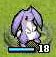# 星之一角

What have you found for these years?

## 2009-12-12

FC++: Functional Programming in C++

==

#### 6 retries:

compile 過，在 runtime 也會掉進 infinite loop；

expansion 還不太知道轉換的推導，不過大體上就是用
lambda 多抽一層出來就對了，也知道最後不會變成 infinite

#define FT std::function
typedef FT＜int(int)＞ F;

struct Untyped {
＿＿Untyped( FT＜F(Untyped)＞ lamb ):lamb_(lamb){}
＿＿F operator()(Untyped xprime) const {
＿＿＿＿return lamb_(xprime);
＿＿}
＿＿FT＜F(Untyped)＞ lamb_;
};

F Z(FT＜F(F)＞ f){
＿＿Untyped temp(
＿＿＿＿[f](Untyped x) -> F {
＿＿＿＿＿＿return f(
＿＿＿＿＿＿＿＿[x](int y) -> int {
＿＿＿＿＿＿＿＿＿＿return x(x)(y);
＿＿＿＿＿＿＿＿}
＿＿＿＿＿＿);
＿＿＿＿});
＿＿return temp(temp);
}

/* in main */

FT＜F(F)＞ almost_fac = [](F f) -> F {
＿＿return [f](int n)->int{
＿＿＿＿if( n == 0 ) return 1;
＿＿＿＿return n*f(n-1);
＿＿};
};
cout << Z(almost_fac)(10) << endl; //3628800

Lin Jen-Shin (godfat) said...

（以下補上 im 上聊到的：）
Y 與 Z 的差別：

Y = λf. (λx. f (x x)) (λx. f (x x))
Z = λf. (λx. f (λy. x x y)) (λx. f (λy. x x y))

eta-conversion
http://en.wikipedia.org/wiki/Eta_expansion#.CE.B7-conversion

f = λx. f x (whenever x does not appear free in f.)

Lin Jen-Shin (godfat) said...

scm said...

Lin Jen-Shin (godfat) said...

\y -> f y 在只吃一個 arg 的狀況下，type 應該是相同的。

\y... -> f y...
y... 則表示有一個以上的 arg forward 過去。